Home > ACC7 > Chapter cc310 > Lesson cc310.1.4 > Problem10-61

10-61.
1. Calculate the value of x. Homework Help ✎

1.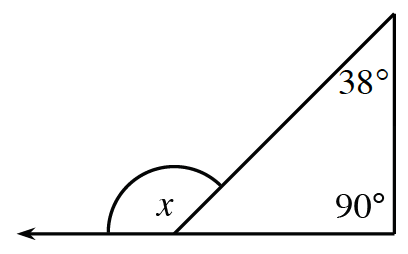2.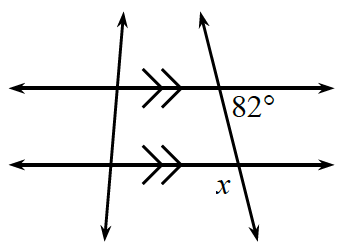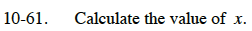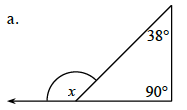Angle x is an exterior angle.

An exterior angle is equal to the sum of the two remote interior angles.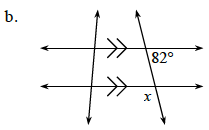Vertical angles are always congruent. The angle that is vertical to x is also supplementary to the given angle.

x = 98°